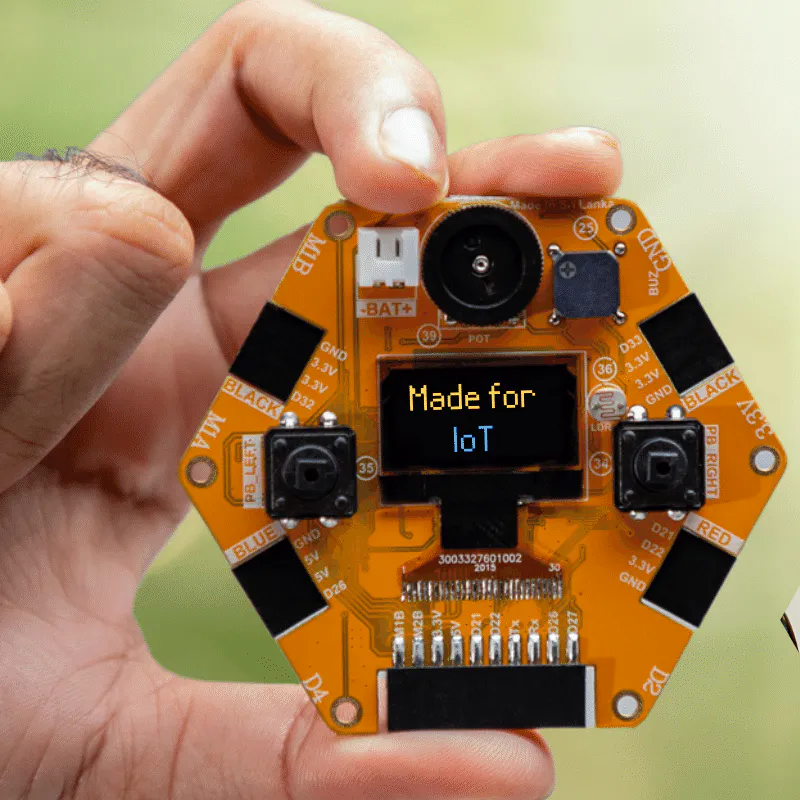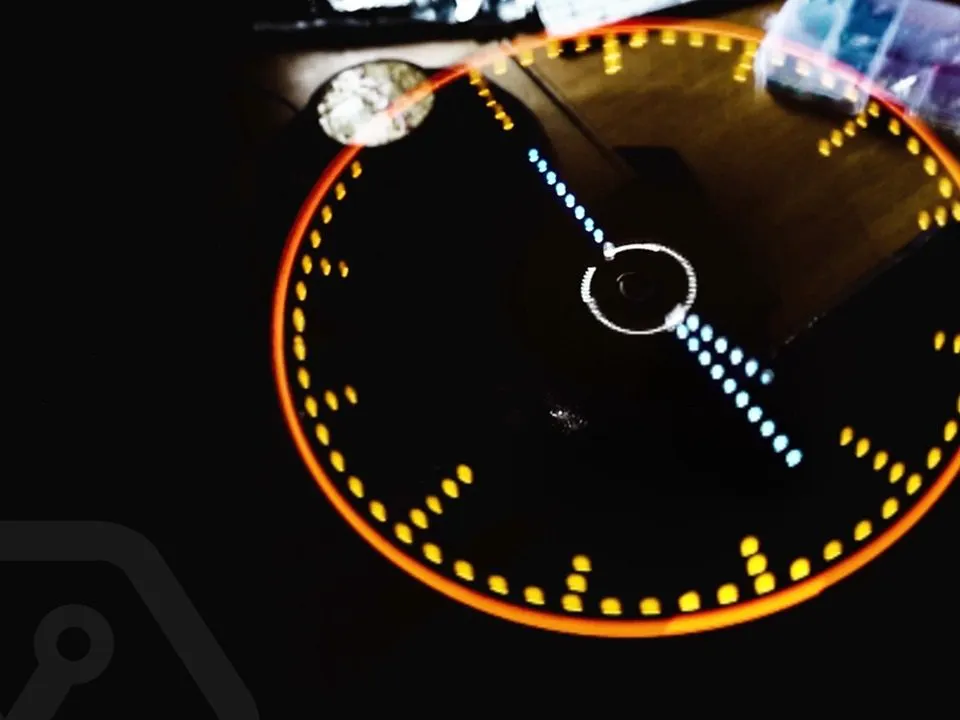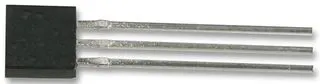Published

# Analog style POV Clock using magicbit-(ESP-32)

In this project, we will make an analog-style POV clock. This is a fun project that you can do very easily at home.

IntermediateShowcase (no instructions)56## Things used in this project

### Hardware components

 magicbit
×1
 17 LEDs of preferred color(Check the circuit diagram).
×1Hall Effect Sensor
×1
 A strong magnet
×1
 12 V motor and a separate source to power it
×1
 A battery to power the circuit.
×1
 A Vero board
×1
 A hot glue gun and maybe some zip ties to securely connect the components
×1

## Code

### Code

Arduino
```// POV LED Clock
// Magicbit
//

int LED1 = 2;
int LED2_3 = 4;
int LED4_5 = 5;
int LED6_7 = 12;
int LED8_9 = 13;
int LED10_11 = 14;
int LED12_13 = 15;
int LED14_15 = 21;
int LED16 = 22;

int sensorPin = 33;

unsigned long previousTime = 0;

byte hours = 12;    // set hours
byte minutes = 15;  // set minutes
byte seconds = 00;  // set seconds

int val;

void setup()
{
pinMode(LED1,OUTPUT);
pinMode(LED2_3,OUTPUT);
pinMode(LED4_5,OUTPUT);
pinMode(LED6_7,OUTPUT);
pinMode(LED8_9,OUTPUT);
pinMode(LED10_11,OUTPUT);
pinMode(LED12_13,OUTPUT);
pinMode(LED14_15,OUTPUT);
pinMode(LED16,OUTPUT);

pinMode(sensorPin,INPUT_PULLUP);

if(hours == 12)
hours = 0;
}

void loop()
{

while (val == LOW)
{
}

if (millis() >= (previousTime))
{
previousTime = previousTime + 1000;
seconds = seconds+1;
if (seconds == 60)
{
seconds = 0;
minutes = minutes+1;
}
if (minutes == 60)
{
minutes = 0;
hours = hours+1;
}
if (hours == 12)
{
hours = 0;
}
}

propeller_posn=30;
n=0;

while(n < 60)
{

drawMinuteMarker();

if ((propeller_posn==0) || (propeller_posn==5) || (propeller_posn==10) || (propeller_posn==15) || (propeller_posn==20) || (propeller_posn==25) || (propeller_posn==30) || (propeller_posn==35) || (propeller_posn==40) || (propeller_posn==45) || (propeller_posn==50) || (propeller_posn==55))
drawHourMarker();

if ((propeller_posn==0) || (propeller_posn==15) || (propeller_posn==30) || (propeller_posn==45))
drawQuarterMarker();

if((propeller_posn == hours*5) || (( propeller_posn == 0 ) && (hours == 0)))
drawHoursHand();

if(propeller_posn == minutes)
drawMinutesHand();

if(propeller_posn == seconds)
drawSecondsHand();

delayMicroseconds(380);     // for LED pixel width   (change the value according to motor speed. Increase for low speed, decrease for high speed motor)

displayClear();

drawInner_Circle();

delayMicroseconds(600);   // for the gap between LED pixels/minutes markers  (change the value according to motor speed. Increase for low speed, decrease for high speed motor)

n++;
propeller_posn++;
if(propeller_posn == 60)
propeller_posn=0;
}

while (val == HIGH)
{
}
}

//=========================

void displayClear()
{
digitalWrite(LED1,LOW);
digitalWrite(LED2_3,LOW);
digitalWrite(LED4_5,LOW);
digitalWrite(LED6_7,LOW);
digitalWrite(LED8_9,LOW);
digitalWrite(LED10_11,LOW);
digitalWrite(LED12_13,LOW);
digitalWrite(LED14_15,LOW);
digitalWrite(LED16,LOW);
}

void drawMinuteMarker()
{
digitalWrite(LED16,HIGH);
}

void drawHourMarker()
{
digitalWrite(LED14_15,HIGH);
}

void drawQuarterMarker()
{
digitalWrite(LED12_13,HIGH);
}

void drawHoursHand()
{
digitalWrite(LED1,HIGH);
digitalWrite(LED2_3,HIGH);
digitalWrite(LED4_5,HIGH);
digitalWrite(LED6_7,HIGH);
}

void drawMinutesHand()
{
digitalWrite(LED1,HIGH);
digitalWrite(LED2_3,HIGH);
digitalWrite(LED4_5,HIGH);
digitalWrite(LED6_7,HIGH);
digitalWrite(LED8_9,HIGH);
}

void drawSecondsHand()
{
digitalWrite(LED1,HIGH);
digitalWrite(LED2_3,HIGH);
digitalWrite(LED4_5,HIGH);
digitalWrite(LED6_7,HIGH);
digitalWrite(LED8_9,HIGH);
digitalWrite(LED10_11,HIGH);
}

void drawInner_Circle()
{
digitalWrite(LED1,HIGH);
delayMicroseconds(30);
digitalWrite(LED1,LOW);
}
```

## Credits

### Magicbit

57 projects • 30 followers
Magicbit is an integrated development platform based on ESP32 for learning, prototyping, coding, electronics, robotics, IoT and more.